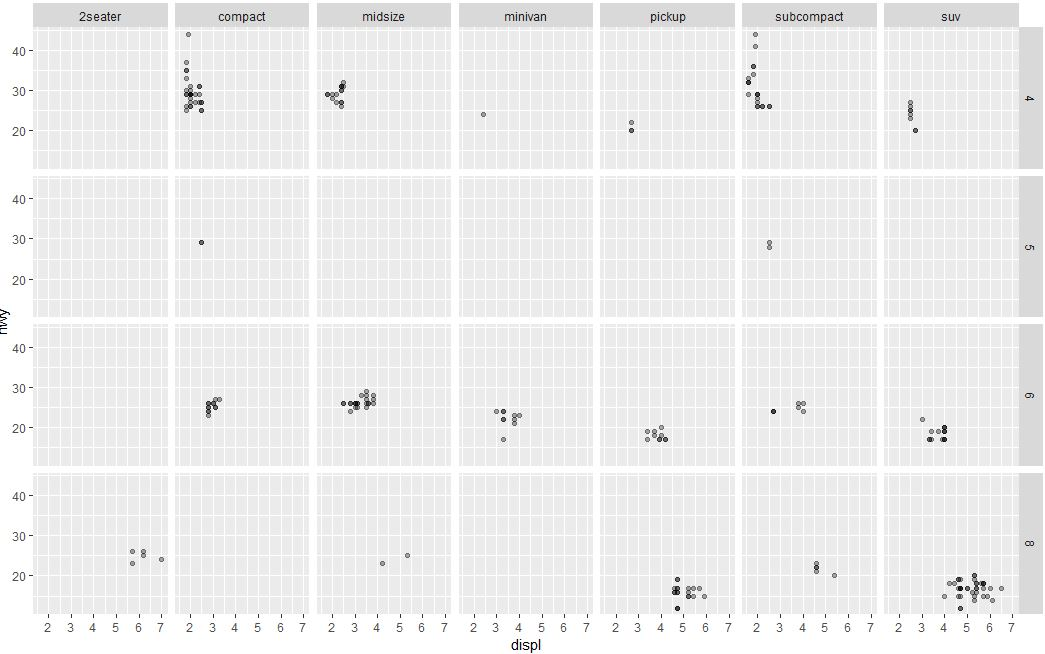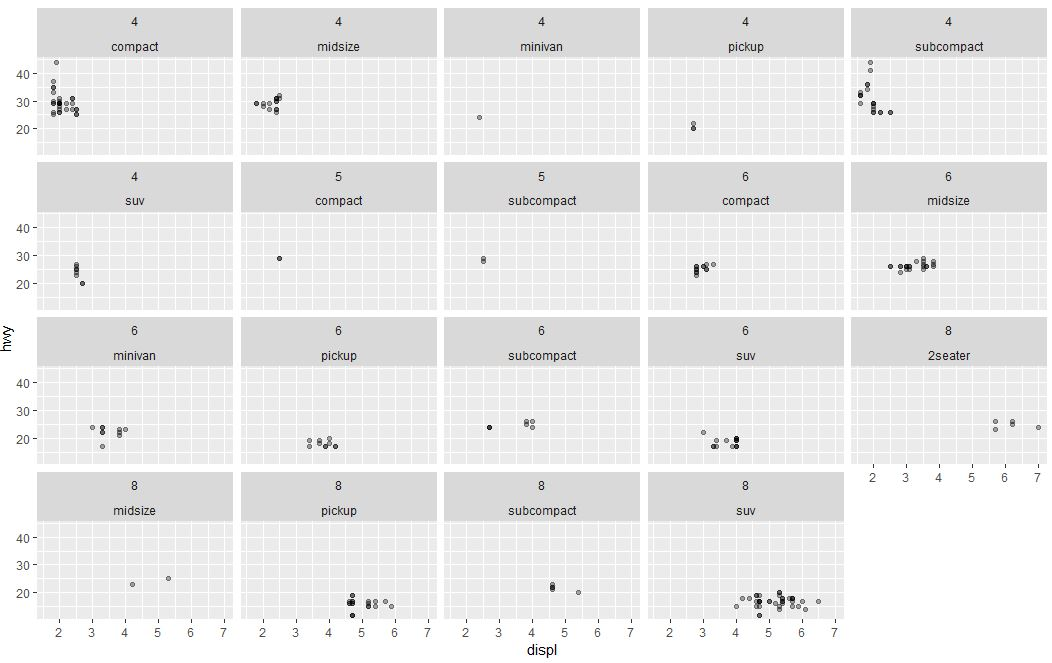2 views

I've been reading the ggplot2 documentation for both functions. I was wondering what were the differences and what would be the right situation for using each function (facet_wrap() and facet_grid()).

library(ggplot2)

p <- qplot(displ, hwy, data = mpg)

p + facet_wrap(~ cyl)

p + facet_grid(~ cyl)

I provide this small example to serve as a starting point. The difference seems to be wrap makes the plots more autonomous and the grid makes one plot altogether.

by
edited

To illustrate the difference lets take a case when you have 2 arguments in facet_grid() or facet_wrap().

facet_grid(x ~ y) will display x*y plots even if some plots are empty. Ex:

library(ggplot2)

g <- ggplot(mpg, aes(displ, hwy))

g + geom_point(alpha=1/3) + facet_grid(cyl~class)There are 4 distinct cyl and 7 distinct class values.

The above displays 4 * 7 = 28 plots, even if some are empty (because some classes do not have corresponding cylinder values, like rows with class="midsize" doesn't have any corresponding cyl="5" value )

facet_wrap(x ~ y) on the other hand, displays only the plots having actual values.i.e.,

g + geom_point(alpha=1/3) + facet_wrap(cyl~class)There are 19 plots displayed now, one for every combination of cyl and class.

If you want to learn more about R programming watch this tutorial on Introduction to Data Science with R